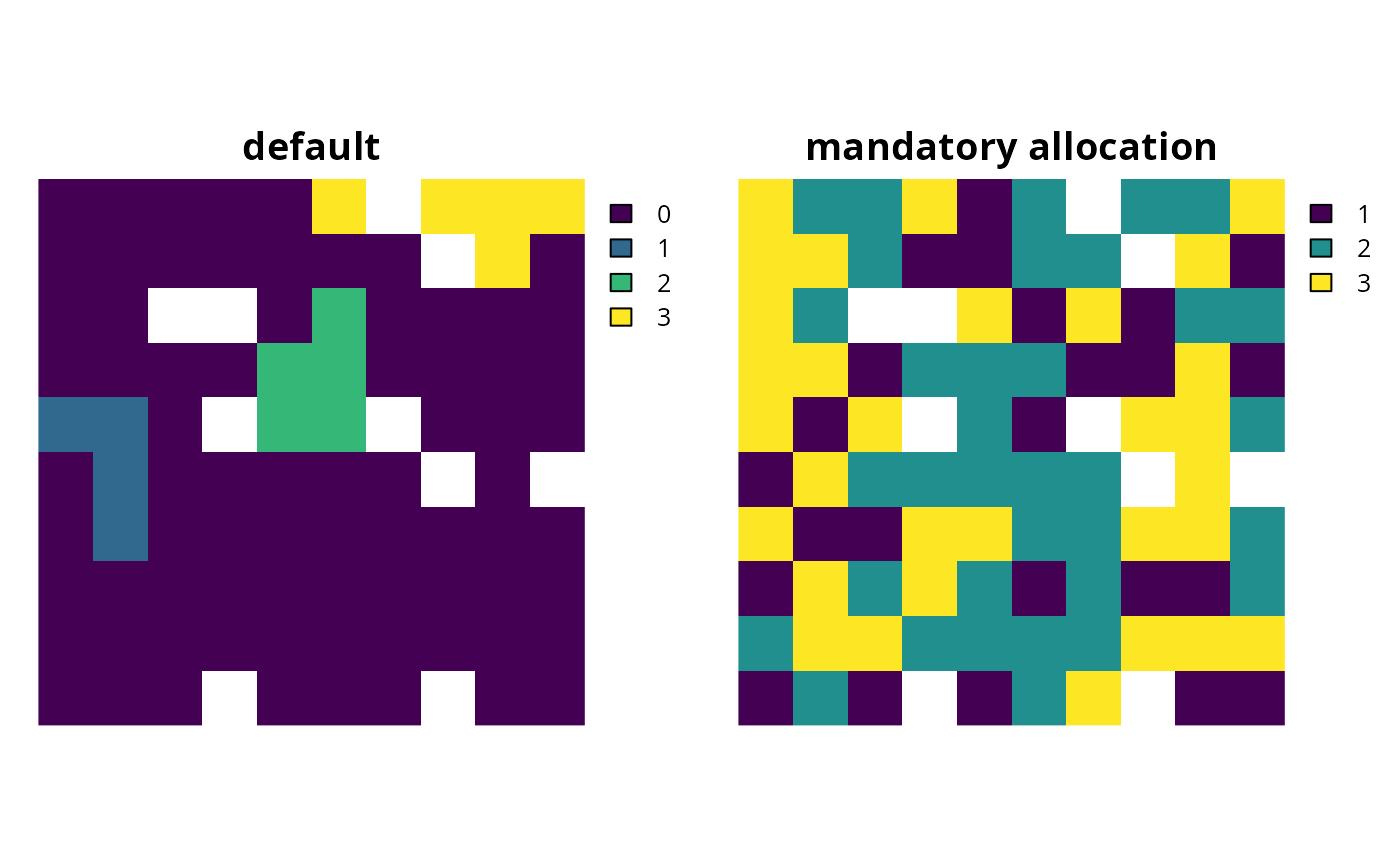Add constraints to ensure that every planning unit is allocated to a management zone in the solution. Note that this function can only be used with problems that contain multiple zones.

# S4 method for ConservationProblem
add_mandatory_allocation_constraints(x)

## Arguments

x problem() (i.e. ConservationProblem) object.

## Value

Object (i.e. ConservationProblem) with the constraints added to it.

## Details

For a conservation planning problem() with multiple management zones, it may sometimes be desirable to obtain a solution that assigns each and every single planning unit to a zone. For example, when developing land-use plans, some decision makers may require that each and every single parcel of land has been allocated a specific land-use type. In other words are no "left over" areas. Although it might seem tempting to simply solve the problem and manually assign "left over" planning units to a default zone afterwards (e.g. an "other", "urban", or "grazing" land-use), this could result in highly sub-optimal solutions if there penalties for siting the default land-use adjacent to other zones. Instead, this function can be used to specify that all planning units in a problem with multiple zones must be allocated to a management zone (i.e. zone allocation is mandatory).

## Examples

# set seed for reproducibility
set.seed(500)

# load data
data(sim_pu_zones_stack, sim_features_zones)

# create multi-zone problem with minimum set objective
targets_matrix <- matrix(rpois(15, 1), nrow = 5, ncol = 3)

# create minimal problem with minimum set objective
p1 <- problem(sim_pu_zones_stack, sim_features_zones) %>%
add_min_set_objective() %>%
add_absolute_targets(targets_matrix) %>%
add_binary_decisions() %>%
add_default_solver(verbose = FALSE)

# create another problem that is the same as p1, but has constraints
# to mandate that every planning unit in the solution is assigned to
# zone
p2 <- p1 %>% add_mandatory_allocation_constraints()
# \dontrun{
# solve problems
s1 <- solve(p1)
s2 <- solve(p2)

# convert solutions into category layers, where each pixel is assigned
# value indicating which zone it was assigned to in the zone
c1 <- category_layer(s1)
c2 <- category_layer(s2)

# plot solution category layers
plot(stack(c1, c2), main = c("default", "mandatory allocation"),
axes = FALSE, box = FALSE)# }